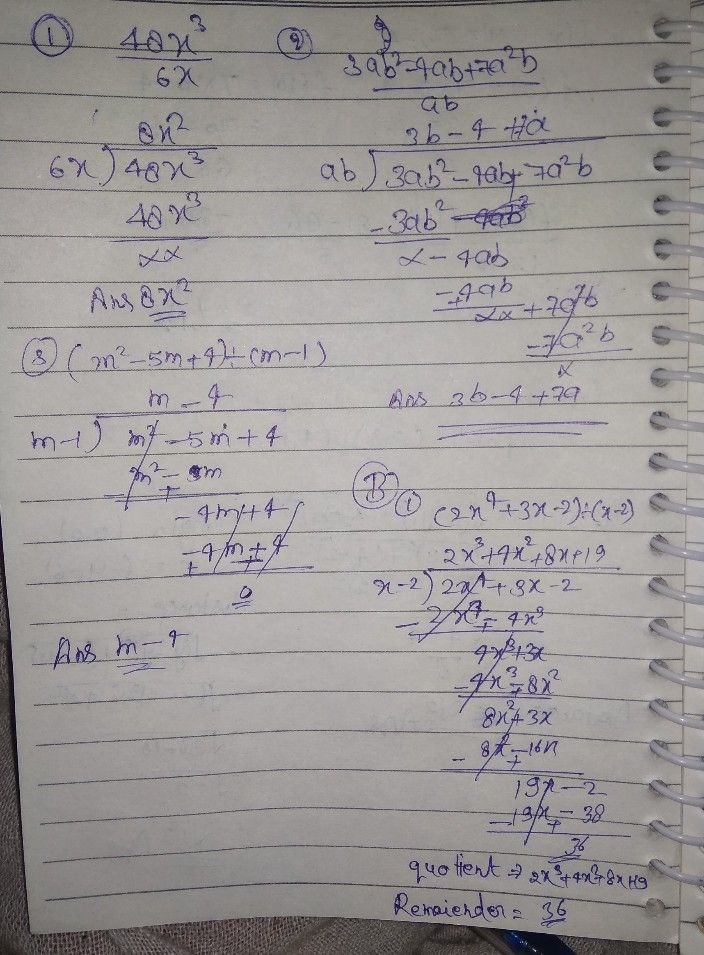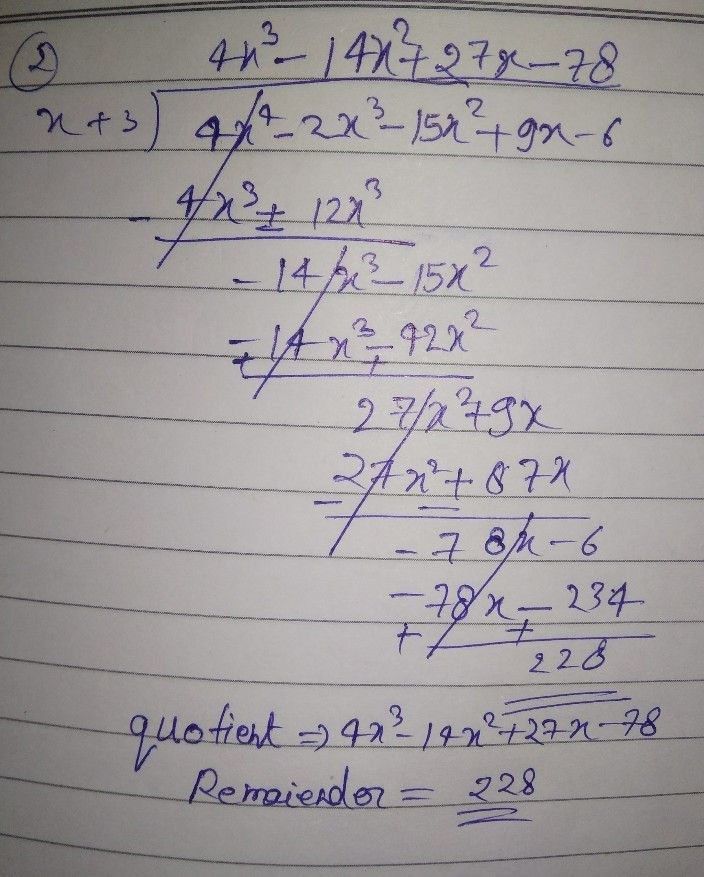Symbol
Problem$Dircctions:$ Do what is asked, $A$ Use long division to simplify cach expression. $1.$ $\dfrac {48x^{1}} {6x}$ $2$ $3ab^{2}-4ab+7x^{2}b$ $ab$ $3$ $\left(m^{2}-5m+4\right)\div \left(m-1\right)$ $B$ Use synthetic division to find the quotient and remainder in each of the $0||o$ $sing$ $1.$ $\left(2x^{4}+3x-2\right)\div \left(x-2\right)$ $2$ $\left(4x^{4}-2x^{3}-15x^{2}+9x-6\right)\div \left(x+3\right)$ PART ?
1st-6th grade
Other
Search count: 108
SolutionQanda teacher - Deepakplease give me review by 5 ?